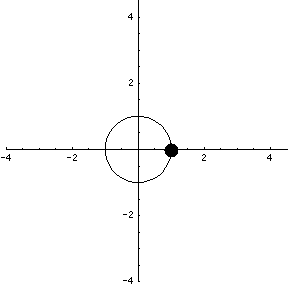# Math Insight

### Image: Parametrization of a circle 2An animation of the parametrization $\dllp(t) = (\cos t, \sin t)$ of the unit circle, where $\dllp(t)$ is the position of a dot at time $t$.

Image file: parametrization_circle_2.gif